Study Materials:
CBSE NOTES ⇒ Class 10th ⇒ Science ⇒ 12. Electricity

## 12. Electricity

### page 2

Connecting resistors to a circuit:

The current through a conductor depends upon its resistance and the potential difference across its ends.

There are two methods of joining the resistors together.

(i) Series: When two or more resistances are joined end to end so that same current flows through each of them. The resistors connecting in such manner is called resistors connected in series.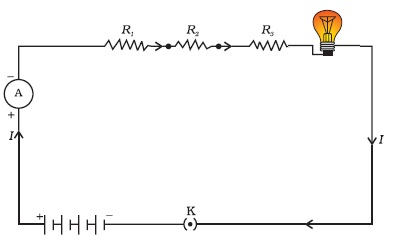Resistors R1, R2 and R3 and a bulb are connected in series

Total Equivalent Resistance in Series:

When we connent resistors R1, Rand R3 in series, the current (I) flowing through the resistance in series will remain same in every part of the circuit, where as the potential difference (V) across each resistor will be diffrerent.

The total potential difference across a combination of resistors inseries is equal to the sum of potential difference across the individual resistors.

Therefore,

V = V1 + V2 + V3          -------------------- (i)

When we apply Ohm's law

V = IR                           --------------------(ii)

So for each part we get;

V1 = I R1     -------------- (iii)
V2 = I R2     -------------- (iv)
V3 = I R3     -------------- (v)

V1 + V2 + V3 = I R1 + I R2 + I R3

V = I (R1 + R2 R3 )                using equation (i)

IR = I (R1 + R2 R3 )              using equation (ii) (V = IR )

which gives

R = R1 + R2 R3

Here R is total equivalent resistance in series which is equal to sums of individual resistances, R1, R2, R3, and is thus greater than any individual resistance.

(ii) Parallel: When two or more resistances are connected across two point so that each of them provides a seperate path for current, The resistors connecting in such manner is called resistors connected in parallel.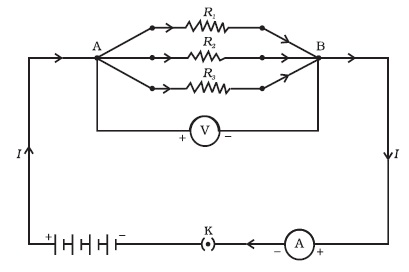Resistors R1, R2 and R3 are connected in parallel.

Total Equivalent Resistance in parallel:

When we connect the resistors R1, R2 and R3 in parallel, The current (I) following in each resistors will be difference but the potential difference (V) across the each resistors will be same.

Therefore,

I = I1 + I2 + I3        -------------- (i)  [current is difference in each resistor]

Let Rp be the equivalent resistance of the parallel combination of resistors.

By applying Ohm’s law to the parallel combination of resistors.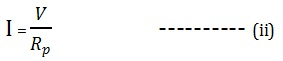On applying Ohm’s law to each resistor, we have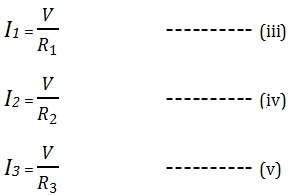adding equation (iii) (iv) and (v)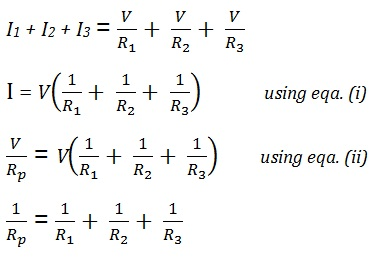Effect on current when resistors are connected in series:

Let, Two resistors R1 and R2 of 3 Ω and 5 Ω are connected in series to a 6 V battery. What will be toatal resistance of circuit and current through the circuit.

Total resistance in circuit

R =  R1 + R2

= 3 Ω + 5 Ω​

= 8 Ω

Now calculating for Current;

I = V/R

= 6/8

= 0.75 A

Effect on current when resistors are connected in parallel:

Same divices connected in parallel then resulting total resistance (R) and current (I)Now calculating for Current;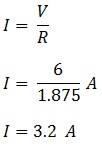See these result in a table

 Resistor Resistance Current Series 8 Ω 0.75 A Parallel 1.875 Ω 3.2 A

Battery: Connections of two or more than two cells is callled battery.

Ohm's Law: the electric current flowing through a metallic wire is directly proportional to the potential difference V, across its ends provided its temperature remains the same. This is called Ohm’s law.

V ∝ I

V ∝ I.constant

V/I = constant

= R

V = IR

Resistance

Heating Effect of electric current:

The chemical reaction within the cell generates the potential difference between
its two terminals that sets the electrons in motion to flow the current through a resistor or a system of resistors connected to the battery.

Some of energy of the source (cell or battery) is used to maintain the current and consumed in usefulwork. Rest of the source energy may be expended in heat to raise the temperature of gadget.

For example, an electric fan becomes warm if used continuously for longer time etc.

On the other hand, if the electric circuit is purely resistive, that is, a configuration of resistors only connected to a battery; the source energy continually gets dissipated entirely in the form of heat. This is known as the heating effect of electric current.

joule's law of heating:

The heat produced in a resistor is directly proportional to the square of current
for a given resistance, directly proportional to resistance for a given current, and  the time for which the current flows through the resistor. This law is called the joule's law of heating.

Joule's law heating is denoted by "H"

H = I2Rt

Establishment of this law in formulla:

Let us suppose that current (I) is flowing through a resistor of resistance
(R) for the time (t). The potential difference across the resistance is (V).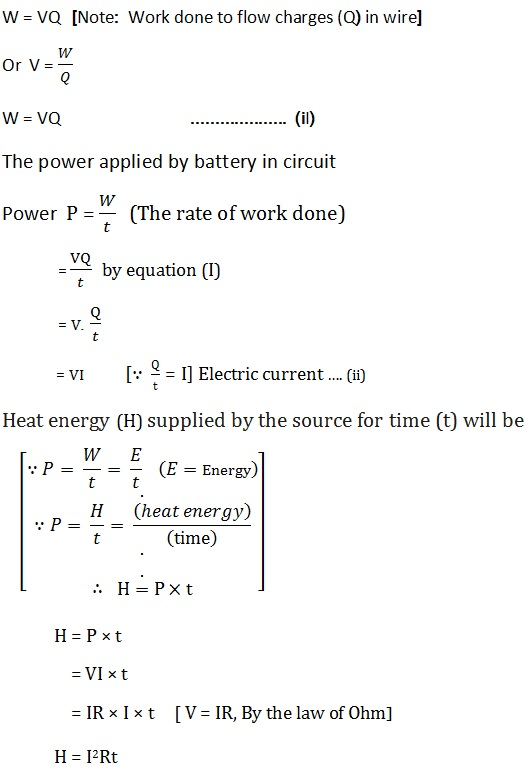The law stated that the heat produced in a resistor is :

(i) Directly proportional to the square of current (I) for a given resistance.

(ii) Directly proportional to resistance (R) for a given current.

(iii) Directly proportional to the time for which the current flows through the resistor.

Other Pages of this Chapter:

Important Study materials for classes 06, 07, 08,09,10, 11 and 12. Like CBSE Notes, Notes for Science, Notes for maths, Notes for Social Science, Notes for Accountancy, Notes for Economics, Notes for political Science, Noes for History, Notes For Bussiness Study, Physical Educations, Sample Papers, Test Papers, Mock Test Papers, Support Materials and Books.Mathematics Class - 11th

NCERT Maths book for CBSE Students.

books

## Study Materials List:

##### CBSE NOTES ⇒ Class 10th ⇒ Science
1. Chemical Reactions and Equations
2. Acids, Bases and Salts
3. Metals and Non-metals
4. Carbon and its Compounds
5. Periodic Classification of Elements
6. Life Processes
7. Control and Coordination
8. How do Organisms Reproduce
9. Heredity and Evolution
10. Light-Reflection and Refraction
11. Human Eye and Colourful World
12. Electricity
13. Magnetic Effects of Electric Current
14. Sources of Energy
15. Our Environment
16. Management of Natural Resources

New Books

See More ...